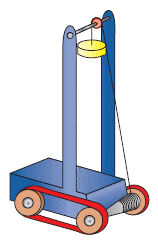# Momentum & Kinetic Energy problem

## Homework Statement

For a technology project, a student has built a vehicle, of total mass 5.70 kg, that moves itself. As shown in the figure below, it runs on two light caterpillar tracks that pass around four light wheels. A reel is attached to one of the axles and a cord originally wound on the reel passes over a pulley attached to the vehicle to support an elevated load. After the vehicle is released from rest, the load descends slowly, unwinding the cord to turn the axle and make the vehicle move forward. Friction is negligible in the pulley and axle bearings. The caterpillar tread does not slip on the wheels or the floor. The reel has a conical shape so that the load descends at a constant low speed while the vehicle moves horizontally across the floor with constant acceleration, reaching final velocity of 2.30i m/s.(a) Does the floor impart impulse to the vehicle? If so, how much?
(b) Does the floor do work on the vehicle? If so, how much?
(c) From where does the final momentum of the vehicle come?
(d) From where does the final kinetic energy of the vehicle come?
(e) Which force causes the forward acceleration of the vehicle?

## Homework Equations

F=ma
Impulse=change in momentum

## The Attempt at a Solution

(a) Yes, 13.11 kg*m/s
(b) No; the direction of the floor's force would be perpendicular to the direction of motion
(c) Not sure. I think it has to be something moving in the opposite direction of the vehicle since v0=0.
(d) The elevated weight since the potential energy is turned into kinetic energy
(e) The axles since they are turning, thereby causing forward acceleration

Thanks in advance to anyone who takes the time to read all that. Am I understanding all these concepts right or are my answers going awry? Also, any hints to (c)?